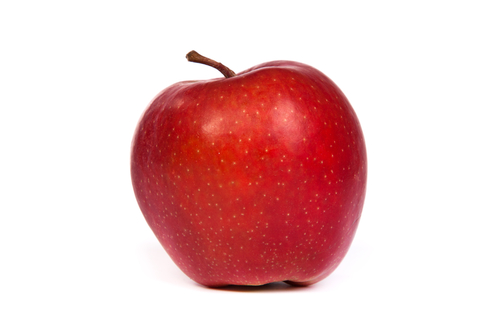#Teacher Note: Names on either side of =¶

Notice that we can use a variable name on either side of the equal sign, `=` (the assignment operator). However, also notice that a variable name is treated differently when found on the left or the right of the equal sign .

For instance, consider this series of assignment statements:

If we think of a variable as a box that holds a value, the meaning of line 1 is fairly obvious: place the value 5 in the box labelled `a`. In line 1, `a` stands for the box associated with the name `a`.

In line 2, however, `a` is found on the right side of `=` . In this case, `a` no longer stands for the box labelled `a` , but rather, the value stored in the box labelled `a` . Therefore, `a + 1` in this case actually means `5 + 1` . In fact, line 2 is executed in these steps:

1. Evaluate the expression `a + 1` to produce a value

2. Replace `a` in the expression with the value stored in `a`, to produce the expression `5 + 1`

3. Evaluate the expression `5 + 1` to produce the value 6

4. Store the value produced (6) in the variable `b`

Of course, the computer performs all these steps for you!

After the first two lines are executed, the print statements will show that `a` will contain 5, while `b` will contain 6.

## Incrementing a variable¶

It’s important to understand the above steps to make sense of the following example. This is a common operation in which the value of a variable is incremented (it’s value is increased by 1):

Line 1 may be straightforward to interpret (assign 5 to the box labelled `a` ), but in line 2, `a` appears twice! This may prove to be confusing to some students. But armed with the above understanding, we can interpret line 2 as follows:

1. Evaluate the expression `a + 1` to produce a value

2. Replace `a` in the expression with the value stored in `a`, to produce the expression `5 + 1`

3. Evaluate the expression `5 + 1` to produce the value 6

4. Store the value produced (6) in the variable `a`

Run the above code to see what the value of the box labelled `a` is when it is printed.

### Self check¶

Consider the following code when trying to solve the problem below:

```distance = 924.7
mpg = 35.5
gallons = distance / mpg
```

The following mixed-up blocks represent the steps that the computer performs to execute these lines. Drag the blocks from the left and put them in the correct order on the right. Click the <i>Check Me</i> button to check your solution.</p>